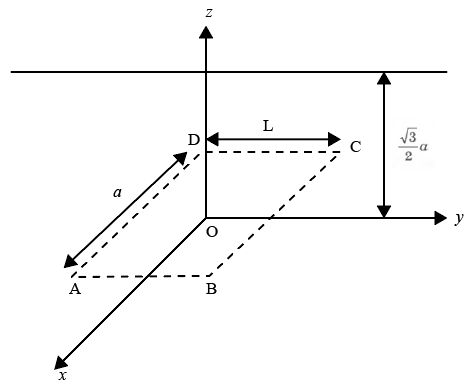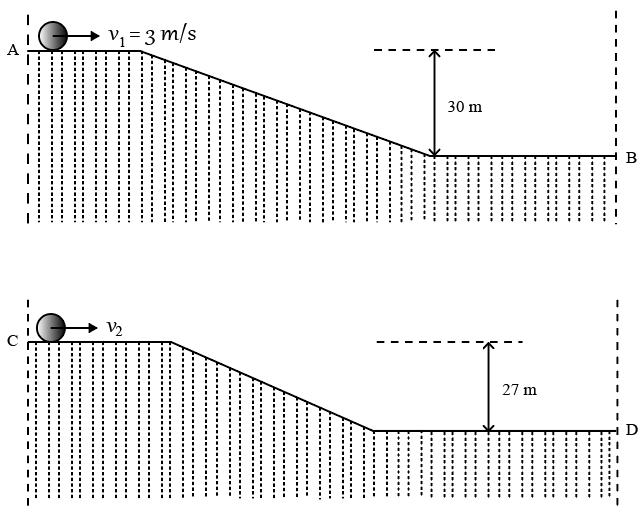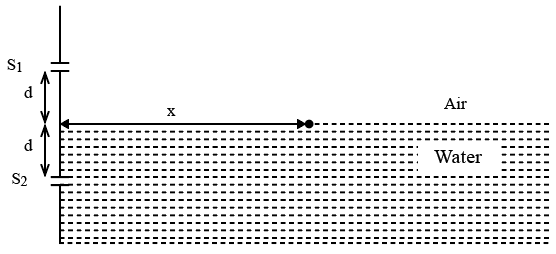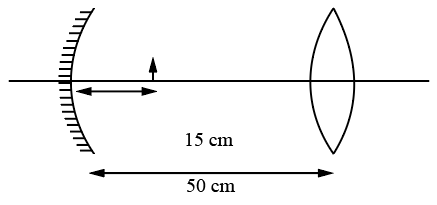Instructions

For the following questions answer them individually

Question 1

# An infinitely long uniform line charge distribution of charge per unit length $$\lambda$$ lies parallel to the y-axis in the y-z plane at $$z = \frac{\sqrt{3}}{2} a$$ (see figure). If the magnitude of the flux of the electric field through the rectangular surface ABCD lying in the x-y plane with its centre at the origin is $$\frac{\lambda L}{n ε_0}$$ ($$ε_0$$ = permittivity of free space), then the value of n isBackspace
789
456
123
0.-
Clear AllQuestion 2

# Consider a hydrogen atom with its electron in the $$n^{th}$$ orbital. An electromagnetic radiation of wavelength 90 nm is used to ionize the atom. If the kinetic energy of the ejected electron is 10.4 eV, then the value of n is (hc = 1242 eV nm)

Backspace
789
456
123
0.-
Clear AllQuestion 3

# A bullet is fired vertically upwards with velocity v from the surface of a spherical planet. Whenit reaches its maximum height, its acceleration due to the planet’s gravity is $$\frac{1}{4^{th}}$$ of its value at the surface of the planet. If the escape velocity from the planet is $$v_{esc} = v\sqrt{N}$$, then the value of N is (ignore energy loss due to atmosphere)

Backspace
789
456
123
0.-
Clear AllQuestion 4

# Two identical uniform discs roll without slipping on two different surfaces AB and CD (see figure) starting at A and C with linear speeds $$v_1$$ and $$v_2$$, respectively, and always remain in contact with the surfaces. If they reach B and D with the samelinear speed and $$v_1 = 3 m/s$$, the $$v_2$$ in m/s is ($$g = 10 m/s^2$$)Backspace
789
456
123
0.-
Clear AllQuestion 5

# Two spherical stars A and B emit blackbody radiation. The radius of A is 400 times that of B and A emits $$10^4$$ times the power emitted from B. The ratio $$\left(\frac{\lambda_A}{\lambda_B}\right)$$ of their wavelengths $$\lambda_A$$ and $$\lambda_B$$ at which the peaks occurin their respective radiation curves is

Backspace
789
456
123
0.-
Clear AllQuestion 6

# A nuclear power plant supplying electrical power to a village uses a radioactive material of half life 7’ years as the fuel. The amountof fuel at the beginningis such that the total power requirement of the village is 12.5% of the electrical power available from the plant at that time. If the plant is able to meet the total power needsof the village for a maximum period of nT years, then the valueof n is

Backspace
789
456
123
0.-
Clear AllQuestion 7

# A Young’s double slit interference arrangement with slits $$S_1$$ and $$S_2$$ is immersed in water (refractive index = $$\frac{4}{3}$$) as shown in the figure. The positions of maxima on the surface of water are given by $$x^2 = p^2m^2 \lambda^2 - d^2$$, where $$\lambda$$ is the wave length of light in air (refractive index = 1), 2d is the separation between theslits and m is an integer. The valueofp isBackspace
789
456
123
0.-
Clear AllQuestion 8

# Consider a concave mirror and a convex lens (refractive index = 1.5) of focal length 10 cm each, separated by a distance of 50 cm in air (refractive index = 1) as shown in the figure. An object is placed at a distance of 15 cm from the mirror. Its erect image formed by this combination has magnification $$M_1$$. When the set-up is kept in a medium of refractive index $$\frac{7}{6}$$, the magnification becomes $$M_2$$. The magnitude $$\mid \frac{M_2}{M_1} \mid$$ isBackspace
789
456
123
0.-
Clear AllQuestion 9

# Consider a Vernier callipers in which each 1 cm on the main scale is divided into 8 equal divisions and a screw gauge with 100 divisions on its circular scale. In the Verniercallipers, 5 divisions of the Vernier scale coincide with 4 divisions on the main scale and in the screw gauge, one complete rotation of the circular scale movesit by two divisions on the linear scale. Then:Question 10

# Planck’s constant h, speed of light c and gravitational constant G are used to form a unit of length L and a unit of mass M. Thenthe correct option(s) is(are)OR